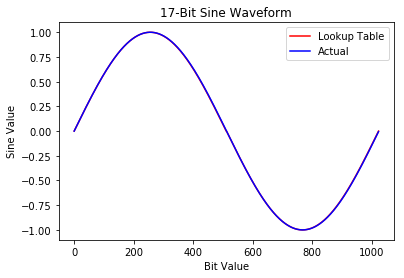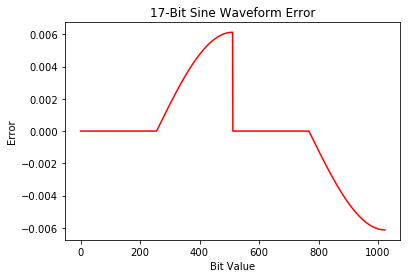# Quarter Wave Debugging

When building a sine lookup table, you can use the symmetry of the sine wave to save lookup table resources. This seemed like a straightforward task but actually had an interesting problem. The sine wave below looks fine, but when compared to the actual waveform a period function appeared in the data.Simulation of the 1/4 wave lookup table vs the real value.Error comparison of the two waveforms above.

The crux ended up being phase quantization. This is shown clearly when the table is reduced to an 8-deep table:

In the initial calculation the sine wave lookup table was based on the equation:

lookup_table(x) = sin((pi/2)*(x/lookup_table_size))

Which resulted in the plots above. While this error probably doesn’t matter much for my application, I was a bit confused by it. I hunted around a bit and found this website which went into detail about the problem and identified a solution which was a half phase slip using the equation

lookup_table(x) = sin((pi/2)((2*x+1)/(lookup_table_size*2)))

This results in a sine wave that’s been shifted, but is otherwise dead on the calculated version!

Which holds as the quarter wave table is expanded to its full scale, 256×16